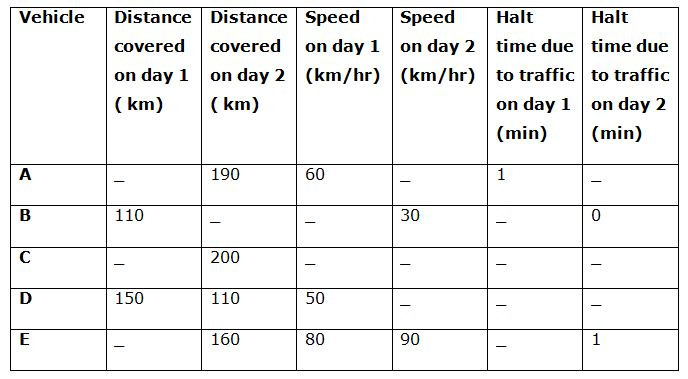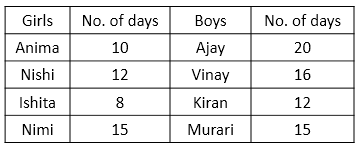# SBI PO Quantitative Aptitude Questions 2019 (Day-32) High Level New Pattern

SBI PO 2019 Notification is about to come and it is the most awaited exam among the aspirants. We all know that new pattern questions are introducing every year in the SBI PO exam. Further, the questions are getting tougher and beyond the level of the candidate’s expectations.

Our IBPS Guide is providing High-Level New Pattern Quantitative Aptitude Questions for SBI PO 2019 so the aspirants can practice it on a daily basis. These questions are framed by our skilled experts after understanding your needs thoroughly. Aspirants can practice these high-level questions daily to familiarize with the exact exam pattern. We wish that your rigorous preparation leads you to a successful target of becoming SBI PO.

[WpProQuiz 5560]

### Click Here for SBI PO Pre 2019 High-Quality Mocks Exactly on SBI Standard

Directions (1 – 5): Read the following information carefully and answer the questions given below:

There are four boys namely Ajay, Vinay, Murari and Kiran and four girls namely Anima, Nishi, Ishita and Nimi in a group and each can complete a piece of work in different number of days. Nishi can complete the piece of work in 12 days. Number of days taken by Murari to complete the work is 25% more than the number of days taken by Nishi to complete the work. Ratio of number of days taken by Murari and Ajay to complete the work is 3:4 respectively. Number of days taken by Ajay to complete the work is 100% more than the number of days taken by Anima to complete the work. Efficiency of Anima is 50% more than the efficiency of Nimi. Respective ratio of the number of days taken by Ajay, Vinay and Ishita is 5:4:2 respectively. Number of days taken by Kiran to complete the work is 50% more than the number of days taken by Ishita to complete the work.

1) Anima and Ishita started the work and left after 2 days. Find the number of days taken by Murari to complete the remaining work.

a) 9 days

b) 33/4 days

c) 8 days

d) 35/4 days

e) None of these

2) If Nimi and Ajay work on alternate days starting with Nimi, find the number of days taken by them to complete the work.

a) 15 days

b) 16 days

c) 10 days

d) 17 days

e) None of these

3) Find the respective ratio of the efficiency of Nishi and efficiency of Vinay.

a) 5:4

b) 4:3

c) 3:2

d) 3:1

e) None of these

4) If Kiran works with half of his efficiency and Murari works with double his efficiency, find the number of days taken by them to complete the work.

a) 130/11 days

b) 140/11 days

c) 120/21 days

d) 110/9 days

e) None of these

5) If all the girls work together, find the number of days taken by them to complete the work.

a) 8/3 days

b) 4 days

c) 7/2 days

d) 2 days

e) None of these

Directions (6 – 10): Study the following graph carefully and answer the given questions6)  A person travelled to reach Pune from Mumbai using vehicle C.  He travelled his journey partly on day 1 and rest on day 2. Total distance between stations is 380 km. On day 1 he travelled at a speed of 10 kmph more than the average speed of entire journey and on day 2 he travelled at a speed of 10 kmph less than the average speed of entire journey. While returning back he decided to reverse the speed on each part of journey find the value of average speed of entire journey if the time taken on return journey is 12 min less than the time taken to reach Pune. (Approximately)

a) 30

b) 55

c) 46

d) 60

e) 54

7) Distance between P and Q is 450 km and two people travelled on day 1. One by vehicle B and other by vehicle C from P to Q. speed of vehicle C is 20 km/h more than that of vehicle B. After travelling 2/3rd distance vehicle A stops for 2 hours and after that remaining distance is covered at 2/3rd of initial speed and reaches at Q. vehicle B after travelling 1/3rd of distance stops for 1 hour and after that increases its speed by 25% and reaches at Q at the same time as the vehicle C. Find the speed of vehicle A.

a) 30

b) 40

c) 60

d) 50

e) Both b) and c)

8) Speed of a man driving vehicle E on day 2. After every 24 minutes he reduces his speed by 5 km/h. Find out the time taken to cover this distance.

a) 4 hrs

b) 2 hrs

c) 3 hrs

d) 1.5 hrs

e) None of these

9) A man starts to travel with A on day 1 in order to reach his in – laws place and reaches 1 hours late. If he travels by vehicle E on day 1 then he reaches 2 hour early. What is the distance between his home and his in-laws place? Also find out the actual time taken to travel the distance. Considering no halt in between and distance covered is same.

a) 11 hrs, 600 km

b) 11 hrs, 720 km

c) 15 hrs, 600 km

d) 12 hrs, 800 km

e) None of these

10) Find the halt time taken by vehicle D on day2. The ratio of halt time on day 1 and day 2 is 5:1. If total time for journey is 5hr 12 min also the time taken on day 2 is 2/3 of day 1

a) 5 min

b) 6 min

c) 7 min

d) 2 min

e) None of these

Direction(1-5):Let, the required number of days = n

2/10 + 2/8 + n/15 = 1

=> n/15 = 1 – 1/5 – ¼

=> n/15 = (20 – 4 – 5)/20

=> n = 15 x 11/20

=> n = 33/4 days

Work completed in 2 days = 1/15 + 1/20

= (4 + 3)/60

= 7/60

Work completed in 2 x 8 days = 7/60 x 8

=> Work completed in 16 days = 56/60

Remaining work = 1 – 56/60

= (60 – 56)/60

= 4/60 = 1/15

= 1/15 which is completed by Nimi in 1 day

Required number of days = 16 + 1 = 17 days

Efficiency of Nishi = 1/12

Efficiency of Vinay = 1/16

Required ratio = 1/12: 1/16 = 4:3

Let, the required number of days = n

n x (1/2 x 1/12 + 2/15) = 1

=> n x (1/24 + 2/15) = 1

=> n x (5 + 16)/120 = 1

=> n = 120/21 days

Let, the required number of days = n

n x (1/10 + 1/12 + 1/8 + 1/15) = 1

=> n x (12 + 10 + 15 + 8)/120 = 1

=> n = 120/45

=> n = 8/3 days

Let the speed of entire journey be x

Distance travelled on day 1

380-200=180

180/(x+10) +200/(x-10)

On return journey

180/(x-10) + 200/(x+10)

Difference of time is 12 min

{180/(x+10) +200/(x-10)} – {180/(x-10) + 200/(x+10)}=12/60

20/(x – 10) – 20/(x + 10) = 1/5

400/(x2 -100) = 1/5

= > x2 – 100 = 2000

= > x2 = 2100

= > x = 46 (Approximately)

The only way to do such question quickly and accurately is to pick options

Let the speed of vehicle B be x

In case 1:

300/(x+20) + 150/((2/3)*(x+20)) +2

In case 2:

150/x + 300/((125/100)*x)+1

As time will be equal so value of case 1 and case 2 will be equal

300/(x+20) + 150/((2/3)*(x+20)) +2 = 150/x + 300/((125/100)*x)+1

Option (a):

X = 30

300/(30+20) + 150/((2/3)*(30+20)) +2 = 150/30 + 300/((125/100)*30)+1

6 + 4.5 + 2 = 5 + 8 + 1

= > 12.5 = 14

LHS ≠ RHS

Option (b):

X = 40

300/(x+20) + 150/((2/3)*(x+20)) +2 = 150/x + 300/((125/100)*x)+1

300/(60) + 150/(2/3*60) + 2 = 150/40 + 300/(125/100*40) + 1

5 + 3.75 + 2 = 3.75 + 6 + 1

= > 10.75 = 10.75

L.H.S = R.H.S

Option (c):

X = 60

300/(x+20) + 150/((2/3)*(x+20)) +2 = 150/x + 300/((125/100)*x)+1

300/60 + 150*3/120 + 2 = 150/40 +300*4/(5*40) + 1

7+ 450/120 = 150/40 + 7

L.H.S = R.H.S

Option (d):

X = 50

300/(x+20) + 150/((2/3)*(x+20)) +2 = 150/x + 300/((125/100)*x)+1

300/70 + 150/(2/3*70) + 2 = 150/50 + 300/(125/100*50) + 1

= > 4.29 + 3.21 + 2 = 3 + 4.8 + 1

= > 9.5 = 8.8

LHS ≠ RHS

For 1st 24 minutes the distance travelled

24/60*90=36 km

For next 24 minutes

24/60*85=34km

For next 24 minutes

24/60*80=32km

For next 24 minutes

24/60*75=30 km

For next 24 minutes

24/60*70=28

So total time taken to cover 160 km is 24*5/60 is 2 hrs

Let the distance travelled be D and time taken be t

According to question

D/60=t+1 = > D = (t + 1) * 60

D/80=t-2 = > D = (t – 2) * 80

As distance covered is same on equating

(t + 1) * 60 = (t – 2) * 80

3t + 3 = 4t – 8

t=11 hrs

D/60=11+1

D=720 km

Time taken on day 1

150/50=3 hrs

Time on day 2

3*2/3=2 hrs

Total time = 5hrs